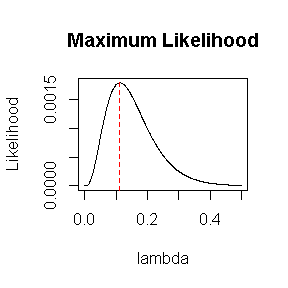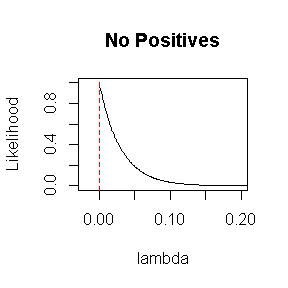# MPN: Most Probable Number for Serial Dilutions

## Introduction

The MPN package computes the Most Probable Number (i.e. microbial density) and other microbial enumeration metrics derived from serial dilutions.

## mpn()

MPN includes the mpn() function to estimate the Most Probable Number (MPN), its variance and confidence interval, and Blodgett’s ( 2, 3, 4 ) Rarity Index (RI).

The user inputs the number of dilutions, number of tubes, number of positive tubes, amount of inocula, confidence level, and confidence interval method.

## Maximum Likelihood Estimation

As discussed in the references, MPN is estimated by maximizing likelihood. Combining the notaton of Blodgett ( 2 ) and Jarvis et al. ( 7 ), we write the likelihood function as:

$L = L(\lambda; x_i, n_i, z_i, i = 1,...,k) = \prod_{i=1}^k \binom{n_i}{x_i} {(1-exp(-{\lambda}{z_i}))} ^ {x_i} {(exp(-{\lambda}{z_i}))} ^ {n_i-x_i}$

where

• $$\lambda$$ is the microbial density (concentration) to be estimated
• $$k$$ is the number of dilution levels
• $$x_i$$ is the number of positive tubes at the $$i^{th}$$ dilution level
• $$n_i$$ is the total number of tubes at the $$i^{th}$$ dilution level
• $$z_i$$ is the amount of inoculum per tube at the $$i^{th}$$ dilution level

As an R function:

#likelihood
L <- function(lambda, positive, tubes, amount) {
binom_coef <- choose(tubes, positive)
exp_term   <- exp(-lambda * amount)
prod(binom_coef * ((1 - exp_term) ^ positive) * exp_term ^ (tubes - positive))
}
L_vec <- Vectorize(L, "lambda")

As is typical of maximum likelihood approaches, mpn() uses the score function (derivative of the log-likelihood) to solve for $$\hat{\lambda}$$, the maximum likelihood estimate (MLE) of $$\lambda$$ (i.e., the point estimate of MPN). However, let’s demonstrate what is happening in terms of the likelihood function itself. Assume we have 10g of undiluted inoculum in each of 3 tubes. Now we use a 10-fold dilution twice (i.e., the relative dilution levels are 1, .1, .01). Also assume that exactly 1 of the 3 tubes is positive at each dilution level:

#MPN calculation
library(MPN)
my_positive <- c(1, 1, 1) #xi
my_tubes    <- c(3, 3, 3) #ni
my_amount   <- 10 * c(1, .1, .01)  #zi
(my_mpn <- mpn(my_positive, my_tubes, my_amount))
#> $MPN #>  0.1118076 #> #>$MPN_adj
#>  0.08724619
#>
#> $variance #>  0.004309218 #> #>$var_log
#>  0.3447116
#>
#> $conf_level #>  0.95 #> #>$CI_method
#>  "Jarvis"
#>
#> $LB #>  0.03537632 #> #>$UB
#>  0.3533702
#>
#> $RI #>  0.005813954 If we plot the likelihood function, we see that $$\hat{\lambda}$$ maximizes the likelihood: my_mpn$MPN
#>  0.1118076
lambda <- seq(0, 0.5, by = .001)
my_L   <- L_vec(lambda, my_positive, my_tubes, my_amount)
plot(lambda, my_L, type = "l", ylab = "Likelihood", main = "Maximum Likelihood")
abline(v = my_mpn$MPN, lty = 2, col = "red")If none of the tubes are positive, the MLE is zero: no_positive <- c(0, 0, 0) #xi (mpn_no_pos <- mpn(no_positive, my_tubes, my_amount)$MPN)
#>  0
L_no_pos <- L_vec(lambda, no_positive, my_tubes, my_amount)
plot(lambda, L_no_pos, type = "l", xlim = c(-0.02, 0.2), ylab = "Likelihood",
main = "No Positives")
abline(v = mpn_no_pos, lty = 2, col = "red")If all of the tubes are positive, then no finite MLE exists:

all_positive <- c(3, 3, 3) #xi
#>  0.1118076
my_mpn$MPN_adj #>  0.08724619 ## Confidence Intervals As discussed in the references, many different confidence intervals (CIs) can be calculated for the Most Probable Number. Currently, mpn() computes a CI using the approach of Jarvis et al. ( 7 ) or the likelihood ratio approach of Ridout (8). However, since these approaches rely on large-sample theory, the results are more reliable for larger experiments. my_positive <- c(1, 1, 1) my_tubes <- c(3, 3, 3) my_amount <- 10 * c(1, .1, .01) mpn(my_positive, my_tubes, my_amount) #Jarvis approach #>$MPN
#>  0.1118076
#>
#> $MPN_adj #>  0.08724619 #> #>$variance
#>  0.004309218
#>
#> $var_log #>  0.3447116 #> #>$conf_level
#>  0.95
#>
#> $CI_method #>  "Jarvis" #> #>$LB
#>  0.03537632
#>
#> $UB #>  0.3533702 #> #>$RI
#>  0.005813954
mpn(my_positive, my_tubes, my_amount, CI_method = "LR")  #likelihood ratio
#> $MPN #>  0.1118076 #> #>$MPN_adj
#>  0.08724619
#>
#> $variance #>  NA #> #>$var_log
#>  NA
#>
#> $conf_level #>  0.95 #> #>$CI_method
#>  "LR"
#>
#> $LB #>  0.02745595 #> #>$UB
#>  0.2975106
#>
#> \$RI
#>  0.005813954

## Rarity Index

As Jarvis ( 7 ) explains, Blodgett’s ( 2, 3, 4 ) Rarity Index is a ratio of two likelihoods. The likelihood in the numerator is for the actual results (i.e., evaluated at the MPN point estimate). The likelihood in the denominator is for the (hypothetical) results that would have given the largest possible likelihood. So RI is larger than 0 and at most 1. Values of RI that are very small are unlikely; therefore, the results should be regarded with suspicion.

## Conclusion

The MPN package is more versatile than static Most Probable Number tables in that the number of tubes can vary across dilution levels, the user can choose any number (or levels) of dilutions, and the confidence level can be changed. Also, the Rarity Index, which quantifies the validity of the results, is included.